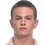# My cryptogram hypothesis

Consider equations, an which ${ A }_{ 1 },{ A }_{ 2 },{ A }_{ 3 },\dots { ,A }_{ n-1 },{ A }_{ n }$ is not necessarily distinct non-zero digits in decimal base and $n, m$ is natural numbers.

1. Prove for $n \ge 5$ that equation below hasn't solution: $\overline { { A }_{ 1 } } +\frac { \overline { { A }_{ 1 }{ A }_{ 2 } } }{ \overline { { A }_{ 2 } } } +\frac { \overline { { A }_{ 1 }{ A }_{ 2 }{ A }_{ 3 } } }{ \overline { { A }_{ 2 }{ A }_{ 3 } } } +\dots +\frac { \overline { { A }_{ 1 }{ A }_{ 2 }{ A }_{ 3 }\dots { A }_{ n-1 }{ A }_{ n } } }{ \overline { { A }_{ 2 }{ A }_{ 3 }\dots { A }_{ n-1 }{ A }_{ n } } } =m$

2. Prove for $n \ge 4$ that equation below hasn't solution: $\overline { { A }_{ 1 } } +\frac { \overline { { A }_{ 1 }{ A }_{ 2 }{ A }_{ 1 } } }{ \overline { { A }_{ 2 } } } +\frac { \overline { { { A }_{ 1 }{ A }_{ 2 }{ A }_{ 3 }{ A }_{ 2 }{ A }_{ 1 } } } }{ \overline { { { A }_{ 2 }{ A }_{ 3 }{ A }_{ 2 } } } } +\dots +\frac { \overline { { { A }_{ 1 }{ A }_{ 2 }\dots { A }_{ n-1 }{ A }_{ n }{ A }_{ n-1 }\dots { A }_{ 2 }{ A }_{ 1 } } } }{ \overline { { A }_{ 2 }\dots { A }_{ n-1 }{ A }_{ n }{ A }_{ n-1 }\dots { A }_{ 2 } } } =m$Note by Ilya Pavlyuchenko
1 year, 3 months ago

This discussion board is a place to discuss our Daily Challenges and the math and science related to those challenges. Explanations are more than just a solution — they should explain the steps and thinking strategies that you used to obtain the solution. Comments should further the discussion of math and science.

When posting on Brilliant:

• Use the emojis to react to an explanation, whether you're congratulating a job well done , or just really confused .
• Ask specific questions about the challenge or the steps in somebody's explanation. Well-posed questions can add a lot to the discussion, but posting "I don't understand!" doesn't help anyone.
• Try to contribute something new to the discussion, whether it is an extension, generalization or other idea related to the challenge.

MarkdownAppears as
*italics* or _italics_ italics
**bold** or __bold__ bold
- bulleted- list
• bulleted
• list
1. numbered2. list
1. numbered
2. list
Note: you must add a full line of space before and after lists for them to show up correctly
paragraph 1paragraph 2

paragraph 1

paragraph 2

[example link](https://brilliant.org)example link
> This is a quote
This is a quote
    # I indented these lines
# 4 spaces, and now they show
# up as a code block.

print "hello world"
# I indented these lines
# 4 spaces, and now they show
# up as a code block.

print "hello world"
MathAppears as
Remember to wrap math in $$ ... $$ or $ ... $ to ensure proper formatting.
2 \times 3 $2 \times 3$
2^{34} $2^{34}$
a_{i-1} $a_{i-1}$
\frac{2}{3} $\frac{2}{3}$
\sqrt{2} $\sqrt{2}$
\sum_{i=1}^3 $\sum_{i=1}^3$
\sin \theta $\sin \theta$
\boxed{123} $\boxed{123}$

Sort by:

Thank you, it is interesting

- 1 year, 2 months ago

Isn't A(1)=1 and A(2)=2 and A(i)=0 for all i>2 a solution for equation 1?

- 1 year, 2 months ago

Whoops, just saw the requirement for non-zero digits.

- 1 year, 2 months ago

@Yajat Shamji, thank you for reporting the spam user. In the future, please tag my account so we can take care of the offending account as soon as possible.

Staff - 1 year, 2 months ago

@Ilya Pavlyuchenko, I don't know how to write cryptograms - I have got one for a problem but I can't write it. Can you give me any help on how to write cryptograms?

- 1 year, 2 months ago

For working in $\LaTeX$ use plugin on browser Daum Equation Editor. You can download it free in Google Chrome Shop.

- 1 year, 2 months ago

Thanks for the alternative!

- 1 year, 2 months ago

No problem!

- 1 year, 2 months ago

@Brilliant Mathematics, I have noticed that in all the profiles of the spam users, the country they come from is Pakistan. Something relevant at all?

- 1 year, 2 months ago

No, I do not think so. But thanks for notifying us!

Staff - 1 year, 2 months ago